Function Repository Resource:

# FactorialSeriesExpansion

Expand a function into a factorial series

Contributed by: Jan Mangaldan
 ResourceFunction["FactorialSeriesExpansion"][expr,z,n] gives the nth-order factorial series expansion of expr in z.

## Details

A factorial series is also known as an inverse factorial series.
The factorial series expansion of a function is expressed in the form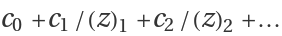, where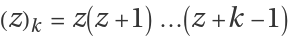is Pochhammer[z,k].
The factorial series expansion can be constructed for any function that can be expanded about the point z=.

## Examples

### Basic Examples (1)

Find the 3rd-order factorial series of e1/x:

 In:=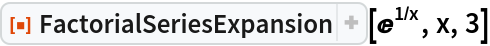Out=In:=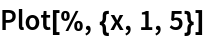Out=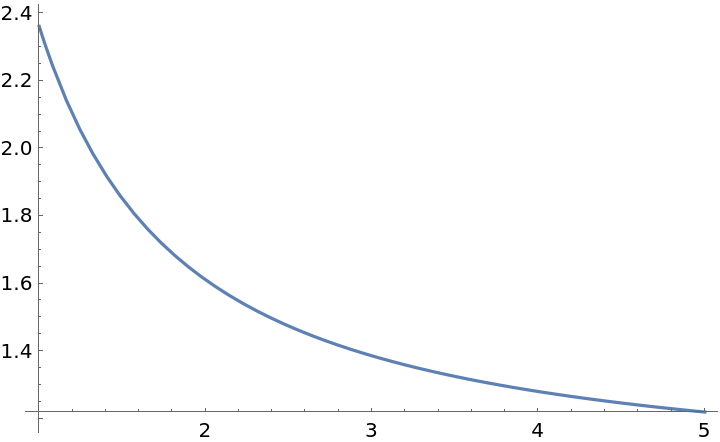### Scope (3)

Find the 5th-order factorial series of the arctangent:

 In:=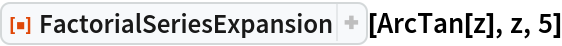Out=Find the 3rd-order factorial series of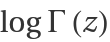:

 In:=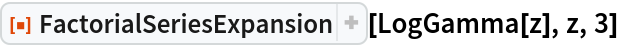Out=Factorial series for a special case of MeijerG:

 In:=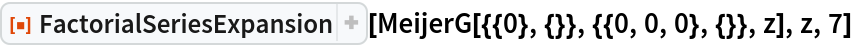Out=### Applications (1)

The factorial series expansion of a function usually has better numerical properties than the corresponding asymptotic series:

 In:=In:=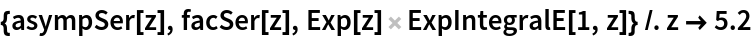Out=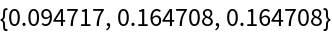In:=Out=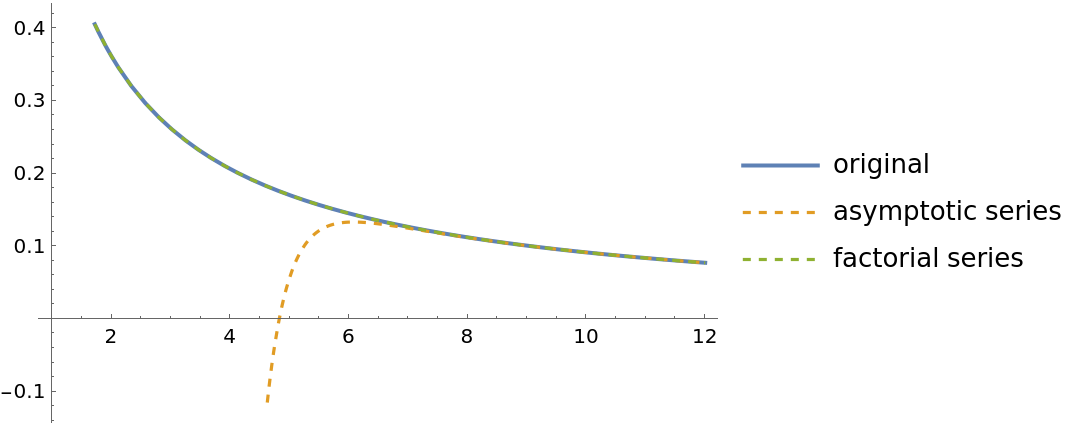### Properties and Relations (1)

The factorial series ofis the function itself:

 In:=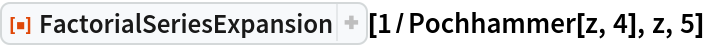Out=## Version History

• 1.0.0 – 22 March 2021